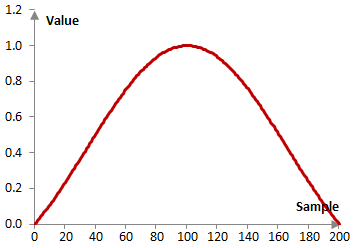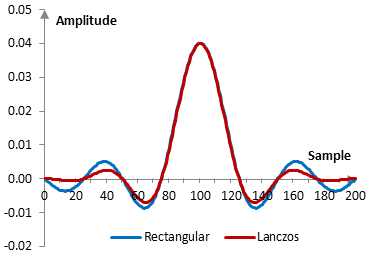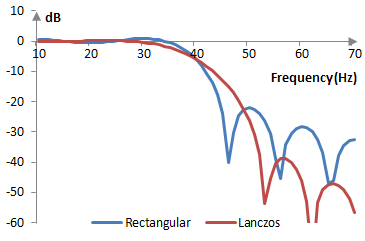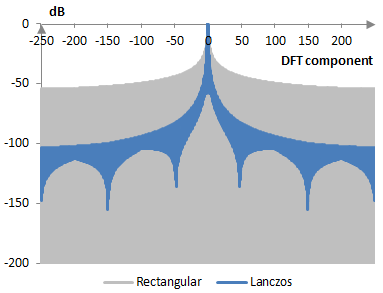# Lanczos window

The Lanczos window coefficients are given by the following formula

$$a(k)=\begin{cases} \frac{\sin(\pi (\frac{2k}{N-1} - 1))}{\pi (\frac{2k}{N-1} - 1)}, k \ne \frac{N-1}{2} \\ 1, k=\frac{N-1}{2} \end{cases}$$

where N is the length of the filter and k = 0, 1, …, N – 1.

Consider a finite impulse response (FIR) low pass filter of length N = 201. The following is the Lanczos window.Given a sampling frequency of 2000 Hz and a filter cutoff frequency of 40 Hz, the impulse response of the filter with a rectangular window (with no window) and with the Lanczos window is as follows.The magnitude response of the same filter is shown on the graph below.## Measures for the Lanczos window

The following is a comparison of the discrete Fourier transform of the Lanczos window and the rectangular window.The Lanczos window measures are as follows.

 Coherent gain 0.59 Equivalent noise bandwidth 1.30 Processing gain -1.14 dB Scalloping loss -1.88 dB Worst case processing loss -3.03 dB Highest sidelobe level -26.4 dB Sidelobe falloff -11.5 dB / octave, -38.3 dB / decade Main lobe is -3 dB 1.26 bins Main lobe is -6 dB 1.74 bins Overlap correlation at 50% overlap 0.272 Amplitude flatness at 50% overlap 0.785 Overlap correlation at 75% overlap 0.733 Amplitude flatness at 75% overlap 0.947

Window

### Filtered HTML

• Freelinking helps you easily create HTML links. Links take the form of [[indicator:target|Title]]. By default (no indicator): Click to view a local node.